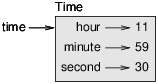# # 第十六章 类和函数

## # 16.1 时间

``````
class Time:
"""Represents the time of day.
attributes: hour, minute, second     """

``````
1
2
3
4
5

``````    time = Time()
time.hour = 11
time.minute = 59
time.second = 30
``````
1
2
3
4Figure 16.1: Object diagram.

## # 16.2 纯函数

``````    def add_time(t1, t2):
sum = Time()
sum.hour = t1.hour + t2.hour
sum.minute = t1.minute + t2.minute
sum.second = t1.second + t2.second
return sum
``````
1
2
3
4
5
6

``````    >>> start = Time()
>>> start.hour = 9
>>> start.minute = 45
>>> start.second =  0
>>> duration = Time()
>>> duration.hour = 1
>>> duration.minute = 35
>>> duration.second = 0
>>> print_time(done)
10:80:00
``````
1
2
3
4
5
6
7
8
9
10
11

``````    def add_time(t1, t2):
sum = Time()
sum.hour = t1.hour + t2.hour
sum.minute = t1.minute + t2.minute
sum.second = t1.second + t2.second
if sum.second >= 60:
sum.second -= 60
sum.minute += 1
if sum.minute >= 60:
sum.minute -= 60
sum.hour += 1
return sum
``````
1
2
3
4
5
6
7
8
9
10
11
12

## # 16.3 修改器

increment 函数，增加给定的秒数到一个 Time 对象，就可以被改写成一个修改器。

``````    def increment(time, seconds):
time.second += seconds
if time.second >= 60:
time.second -= 60
time.minute += 1
if time.minute >= 60:
time.minute -= 60
time.hour += 1
``````
1
2
3
4
5
6
7
8

## # 16.4 原型与规划

``````    def time_to_int(time):
minutes = time.hour * 60 + time.minute
seconds = minutes * 60 + time.second
return seconds
``````
1
2
3
4

``````
def int_to_time(seconds):
time = Time()
minutes, time.second = divmod(seconds, 60)
time.hour, time.minute = divmod(minutes, 60)
return time
``````
1
2
3
4
5
6

``````
seconds = time_to_int(t1) + time_to_int(t2)
return int_to_time(seconds)
``````
1
2
3
4

## # 16.5 调试

``````
def valid_time(time):
if time.hour < 0 or time.minute < 0 or time.second < 0:
return False
if time.minute >= 60 or time.second >= 60:
return False
return True
``````
1
2
3
4
5
6
7

``````    def add_time(t1, t2):
if not valid_time(t1) or not valid_time(t2):
raise ValueError('invalid Time object in add_time')
seconds = time_to_int(t1) + time_to_int(t2)
return int_to_time(seconds)
``````
1
2
3
4
5

``````
assert valid_time(t1) and valid_time(t2)
seconds = time_to_int(t1) + time_to_int(t2)
return int_to_time(seconds)
``````
1
2
3
4
5

assert 语句是很有用的，可以用来区分条件语句的用途，将 assert 这种用于检查错误的语句与常规的条件语句在代码上进行区分。

## # 16.6 Glossary 术语列表

prototype and patch: A development plan that involves writing a rough draft of a program, testing, and correcting errors as they are found.

designed development: A development plan that involves high-level insight into the problem and more planning than incremental development or prototype development.

pure function: A function that does not modify any of the objects it receives as arguments. Most pure functions are fruitful.

modifier: A function that changes one or more of the objects it receives as arguments. Most modifiers are void; that is, they return None.

functional programming style: A style of program design in which the majority of functions are pure.

invariant: A condition that should always be true during the execution of a program.

assert statement: A statement that check a condition and raises an exception if it fails.

assert 语句：一种检查错误的语句，检查一个条件，如果不满足就抛出异常。

## # 16.7 练习

### # 练习2

datetime 模块提供了一些 time 对象，和本章的 Time 对象很相似，但前者提供了更多的方法和运算符。读一读[这里的文档] Here吧。

1. 用 datetime 模块来写一个函数，获取当前日期，然后输出今天是星期几。

2.写一个函数，要求输入生日，然后输出用户的年龄以及距离下一个生日的日、时、分、秒数。

3.有的两个人在不同日期出生，会在某一天，一个人的年龄是另外一个人年龄的两杯。这一天就叫做他们的双倍日。写一个函数，接收两个生日，然后计算双倍日。

1. 再来点有挑战性的，写一个更通用的版本，来计算一下一个人的年龄为另外一个人年龄 n 倍时候的日期。